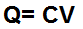﻿ Capacitance Charge Calculator ﻿# Capacitor Charge CalculatorpF nF µF F

V

0.0000000000C

The Capacitor Charge Calculator calculates the charge of a capacitor with a a capacitance, of C, and a voltage of V, according to the formula Q=CV.

A user just enters in the two parameters, capacitance, C, and voltage, V, and the resultant charge is automatically computed. The value of the resultant is expressed in unit coulombs (C).

The charge on a capacitor's terminals is a quantitative measure of the amount of electrons which are on the plates (terminals) of the capacitors. The more electrons, the greater the charge. The less electrons, the lesser the charge. Charge is important because many devices, such as flash cameras, operate on the charge which a capacitor attains.

Related Resources

﻿